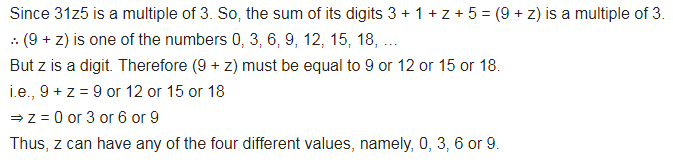# 31z5 is a multiple of 3, where z is a digit, what might be the values of z?

31z5 is a multiple of 3, where z is a digit, what might be the values of z?